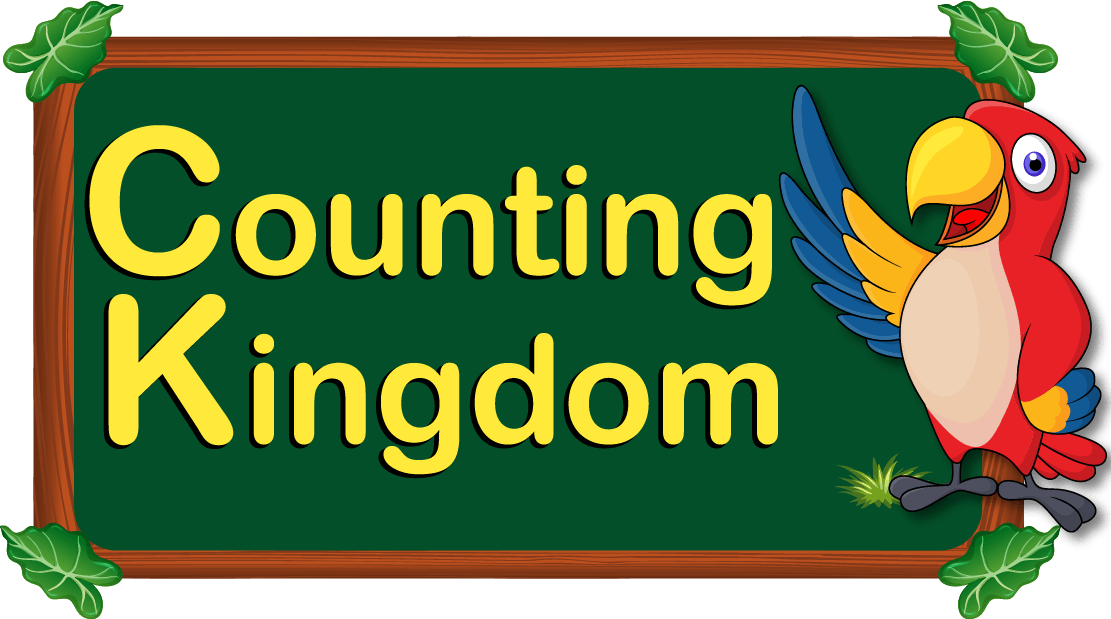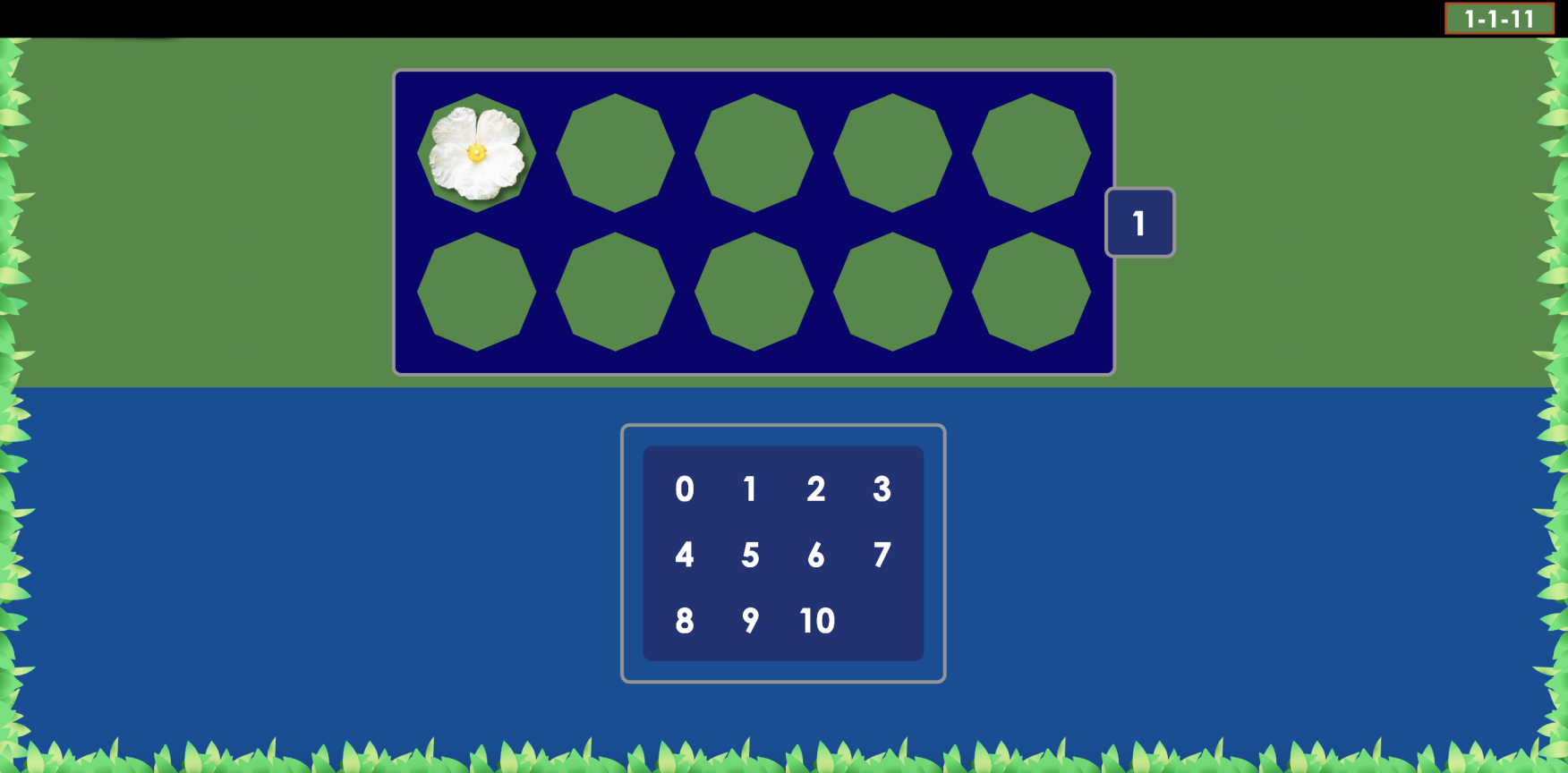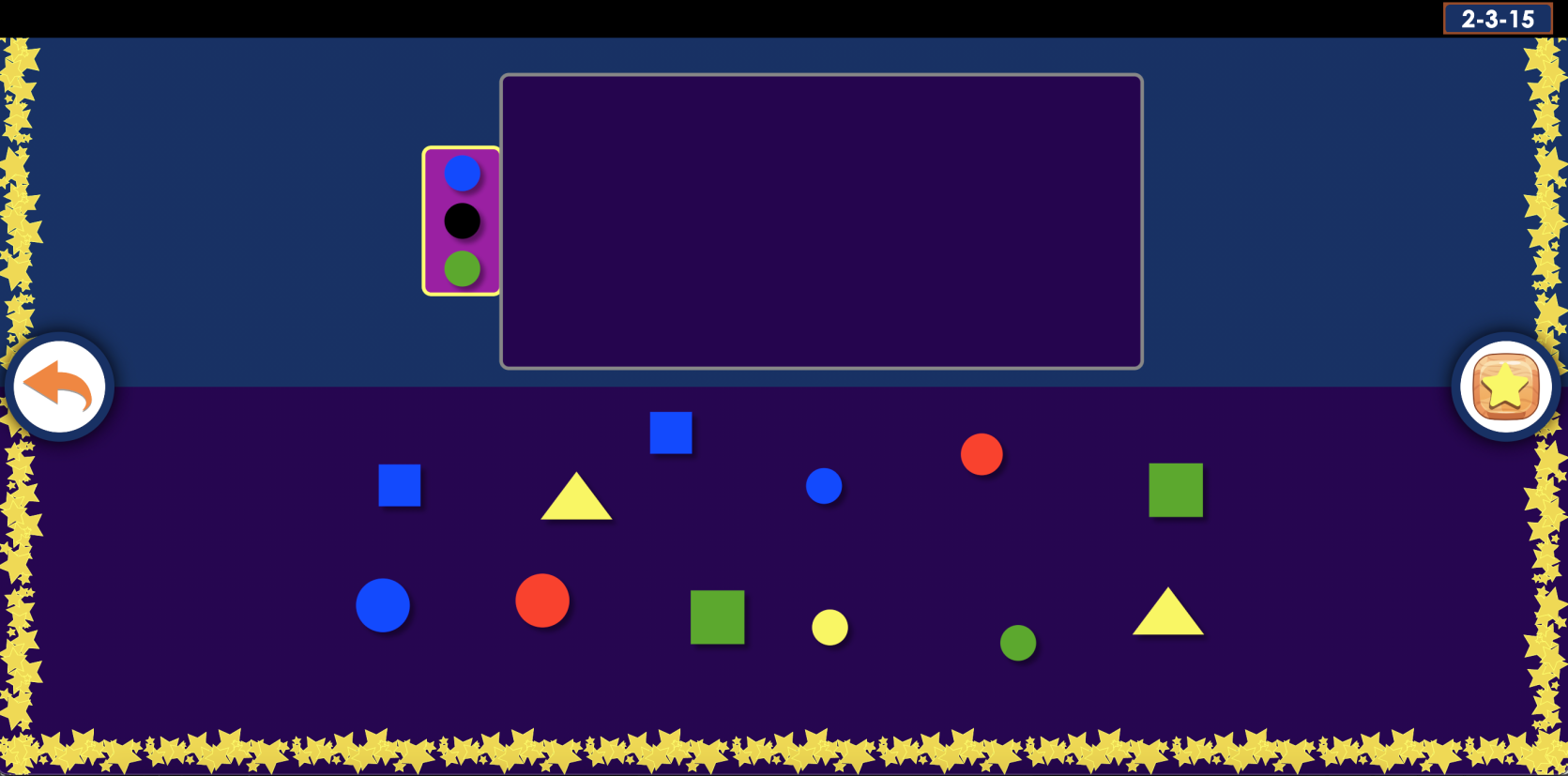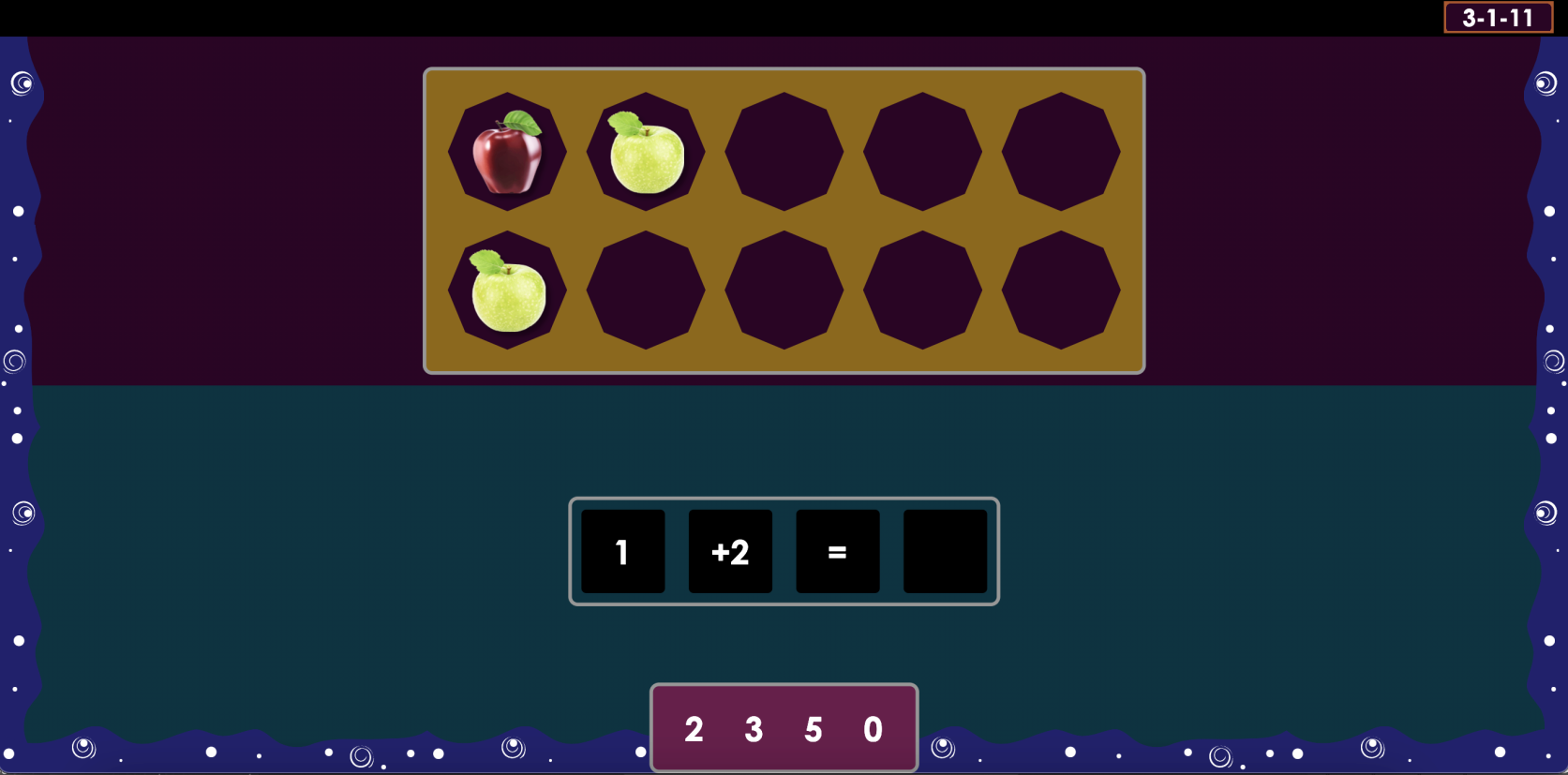Works with Windows, Mac, iPad, Android, Chromebooks

“We found Counting Kingdom to be easy to use, and fun. I really like that it works with a ten frame and creates that visual concepts - this was the main reason I wanted to review the program. Ten frames are such a great tool for math concepts using numbers 1-10.” - Meghan Wright, parent

Counting Kingdom is an online introductory math program that teaches the foundations of math.Counting Kingdom program uses a rich array of techniques that teaches students to:

• recognize, count, and understand the meaning of numbers up to 10
• calculate the number of objects in a group
• understand that a particular number of objects has a fixed value despite the size or nature of those objects
• understand geometric shapes and be able to sort objects by shape
• follow a sequence of commands involving math concepts
• comprehend key math concepts such as “same as,” “all”, “another one” and “the right number.”

Along with the teaching of core ideas in math, the program enhances a student’s conceptual and reasoning abilities.

Another key goal of the program is to teach students to understand the variety of linguistic forms in which math concepts can appear (e.g., the request to “calculate a number of objects” can appear as:

• “How many are here?”
• “Count these.”
• “Give me X (e.g., 4) of these.”
• “Write the number that shows how many are here.” etc.)The program is divided into 3 levels.

• In Level 1 students gain a conceptual understanding of numbers 1 through 10.• In Level 2, higher-level math-related concepts are introduced such as all, same number, other, etc. These concepts entail a level of generalization that is independent of specific numbers but applicable to all numbers.• In Level 3, a range of memory demands are introduced. Memory is critical in enabling students to use what they have learned in the past so that they can avoid “starting from scratch” with each and every task.As a student progresses through the program, earlier items from prior levels are maintained. The steady intermixing of what has been learned with new material reinforces the earlier material and empowers students with a sense of mastery.

Counting Kingdom uses a “dot sheet” - a unique tool that provides a visual aid that helps organize a student’s counting and perception of number.

Requirements:

Appropriate for children 3+ who are able to use a tablet or a computer and mouse.

Counting Kingdom is \$49.99 for one year access